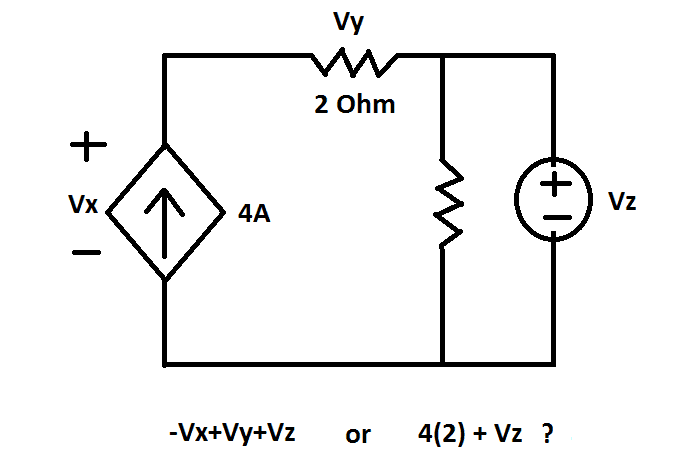# Independent Current Source In Series With Resistor

## Homework Statement

Write the KVL Equation for the outer loop## The Attempt at a Solution

The question above is a sample I have made for such a condition. The goal is not to find the value, but only write the correct equation. If an independent current source is connected in series with a resistor, should I write the voltages across the resistor and the current source separately in the KVL equation or should I simply apply Ohms law V=IR and write the result in the KVL equation?
The first equation that I have made is -Vx+Vy+Vz=0. The other equation that I have made is 4(2)+Vz=0, where 4(2) is the resistance of the resistor multiplied by the current of the independent current source. Which one of these is the right one?

phinds
Gold Member
All in all, this is an EXTREMELY silly circuit from a practical point of view but does demonstrate some interesting things.

As far as the rest of the circuit is concerned, the series resistor is irrelevant and can be just as easily removed from the circuit. Take the current source and the series resistor and draw a box around them. What comes out of the box is 4amps, regardless of whether or not there is a series resistor in the box. That is, the box is just a 4 amp current source, just like the 4 amp source was before you drew the box.

Now, if you DO want to look at the current source and the resistor and make the rest of the circuit a black box, then the voltage across the current source becomes a function of both the resistor and what's in the black box. The resistor will drop 8 volts and then the voltage across the current source is 8 volts less than whatever the voltage drop is across the rest of the circuit.

In this case you have a specific "rest of the circuit" that includes a resistor in parallel with a voltage source. Like the resistor that is in series with the current source, this resistor is irrelevant to the rest of the circuit. It has Vz volts across it. Period. If you want to figure out the current through the voltage source then you DO have consider the resistor because it sinks some of the current provided by the rest of the circuit and the voltage source has to sink the rest.

NascentOxygen
Staff Emeritus
Write the KVL Equation for the outer loop
I like to see voltage arrows across components before forming or confirming KVL equations.

The voltage arrow belonging across both sources is apparent from the + and - signs on each, though nevertheless it is still helpful to draw in each arrow. How you draw the arrow for Vy determines the sign it has in the KVL equation. It is not always going to be "obvious" which way the voltage arrow should point.

The first equation that I have made is -Vx+Vy+Vz=0.
That looks like it's right.

Thanks